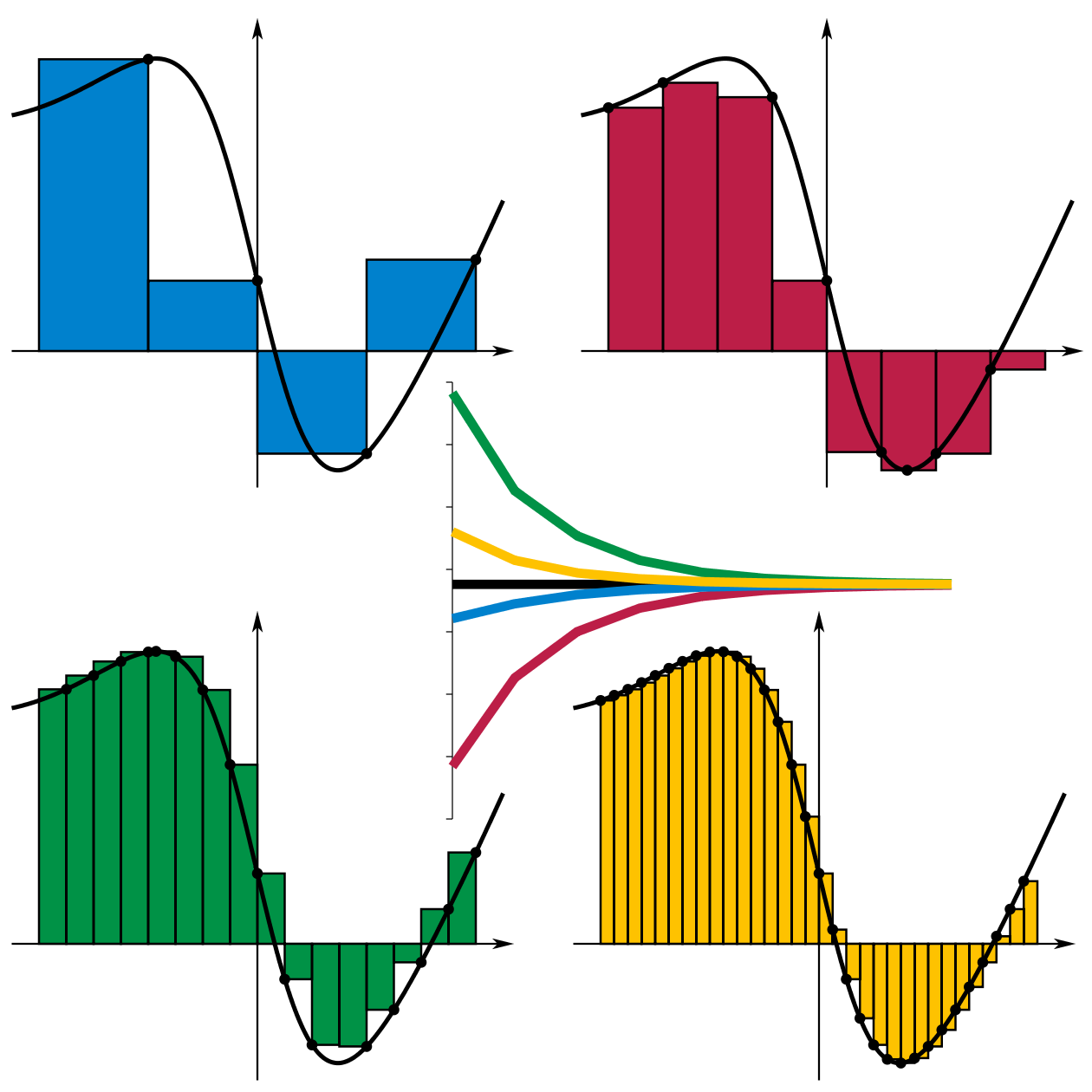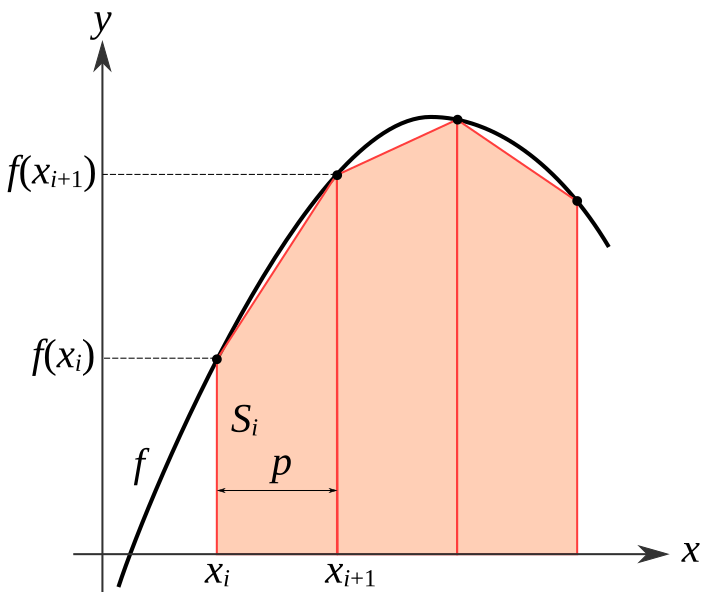# Numerical integration in R part I

## Instructions:

Use the left and right arrow keys to navigate the presentation forward and backward respectively. You can also use the arrows at the bottom right of the screen to navigate with a mouse.

FAIR USE ACT DISCLAIMER:
This site is for educational purposes only. This website may contain copyrighted material, the use of which has not been specifically authorized by the copyright holders. The material is made available on this website as a way to advance teaching, and copyright-protected materials are used to the extent necessary to make this class function in a distance learning environment. The Fair Use Copyright Disclaimer is under section 107 of the Copyright Act of 1976, allowance is made for “fair use” for purposes such as criticism, comment, news reporting, teaching, scholarship, education and research.

## Outline

• The following topics will be covered in this lecture:
• Basic ideas of numerical integration
• Newton-Cotes
• The trapezoid rule

## Basic ideas of numerical integration

• In basic calculus, we learn about various types of functions that have easy-to-solve integrals with closed form, e.g.,

• Polynomials follow simple rules in terms of anti-derivatives and definite integrals;
• Trigonometric functions have many nice relationships that allow us to find anti-derivatives;
• certain forms of equations allow substitutions or integration by parts in order to re-write integrals into simple forms.
• However, there are far more integrals that cannot be solved this way than can be solved by hand.

• One important example is the Gaussian function, $f(x) = e^{-x^2}$ which does not have a simple closed form, and it is non-trivial to show that this integrates to $$\sqrt{\pi}$$.
• Generally, integrals have no closed form in terms of evaluating anti-derivatives;
• we can instead appeal to the construction of integrals directly to get some insight into how we can perform integration by computer.

### Riemann sums

• Recall, when we define a Riemann sum, this is very similar to how a density estimator like a histogram or kernel density estimator works.

• We will suppose that there is some function $f:[a,b] \rightarrow \mathbb{R}$ that we wish to integrate over the range above.

• If $$f(x)$$ can be evaluated at any given point $$x\in [a,b]$$, we can take a partition of the interval $$[a,b]$$ as follows:

• Let $$a = x_0 < x_1 < \cdots < x_n=b$$;
• We define sub-intervals of $$[a,b]$$ with the above points defining the endpoints, i.e.,

\begin{align} I_i = [x_{i-1}, x_i] & & \cup_{i=1}^n I_i = [a,b] \end{align}

• Finally, for each $$i$$, we choose a representative point for the interval $$x_i^\ast\in I_i$$ that will be used to evaluate the weight given to the interval.
• Let us finally write the length of the $$i$$-th interval as $$\Delta_i = x_{i} - x_{i-1}$$, and denote the collection of all the intervals and the representative points as $$\mathcal{I}_n$$;

• then a Riemann sum on this partition is defined as,

\begin{align} S\left(\mathcal{I}_n\right) = \sum_{i=1}^n f\left(x_i^\ast\right) *\Delta_i \end{align}

### Examples of Riemann sumsCourtesy of I, KSmrq via Wikimedia Commons CC BY-SA

• To the left, we see several different interpretations of Riemann sums for a given function, represented by the black curve:
• We vary the size of the partition $$n$$, as well as the choice of the $$x_i^\ast$$ representative points.
• Top left: we see the right-endpoint method, where $$x_i^\ast = x_i$$ for all $$i$$.
• Top right: wherever $$f(x)$$ attains its minimum value over the range $$I_i$$ is chosen as $$x^\ast_i$$.
• Bottom left: wherever $$f(x)$$ attains its maximal value over the range $$I_i$$ is chosen as $$x^\ast_i$$.
• Bottom right: we see the the left-endpoint method, where $$x^\ast_i = x_{i-1}$$ for all $$i$$.
• The plotted lines then show how fast the discrete approximations converge to the true value of the integral as we take smaller widths $$\Delta_i$$ and more sub-intervals in the partition $$\mathcal{I}_n$$ as $$n\rightarrow \infty$$.
• Most numerical integration follows a pattern like this, in which we will look for clever ways to find a representative value for a partition, so that we can get the true value asymptotically in the refinement of the partition.

• Although the method of Riemann sums discussed is relatively simple to implement in a computer language, it suffers from disadvantages like histograms do.

• Specifically, the Riemann sums discussed earlier have a very slow rate of convergence;
• i.e., you must take a very fine partition of the interval, and evaluate $$f$$ many times, to get accurate results.
• Like we considered with the kernel densities, we may instead use a smoothing function that will give weight to values of $$f(x)$$ away from the representative point $$x_i^\ast$$ for the interval $$I_i$$.

• For reasons that won't be considered directly in this class, polynomials can make very good approximations of arbitrary functions within certain bounds.

• Polynomials are also very easy to integrate, so that if we can approximate $$f$$ well by some polynomial $$p_n(x)$$, we can get a faster converging sum by this approximation.

• This idea is known as quadrature.

## Newton-Cotes rule

• Let's suppose that a partition $$I_n$$ of $$[a,b]$$ is given with endpoints $$a=x_0 < \cdots < x_n = b$$.

• We will call the class of basis polynomials $$P_n$$ which will be a collection of all polynomials $$p_n \in P_n$$ for which \begin{align} p_n(x) = \sum_{i=1}^n x^i c_i & & p_n(x_i) = f(x_i) \end{align} for some coefficient values $$c_i$$.

• The goal then is to choose some such polynomial $$p_n$$ that gives a good approximation of $$f(x)$$ over each sub-interval in the partition.

• We will define a generic interpolation polynomial as follows:

\begin{align} L_k(x) = \prod_{i=0,i\neq k}^n \frac{x - x_i}{x_k - x_i} \end{align}

where $$x_i$$ are the endpoints of the partition and the product goes over all terms $$x_i$$ except for $$x_k$$ to avoid a division by zero.

• Specifically, when we evaluate $$L_k(x_k)$$ we get the value $$1$$ by all numerators equaling the denominators.
• However, at any other $$x_i$$, $$L_k(x_i) = 0$$ for all $$i \neq k$$.

### Newton-Cotes rule

• Using the interpolation polynomials, we construct the Lagrange basis polynomial $$p_n$$ as follows:

\begin{align} p_n(x) = \sum_{i=1}^n f(x_i)L_i(x) \end{align}

so that this evaluates to $$f(x_i)$$ at each endpoint in the partition, but it interpolates between the points with better accuracy than the flat Riemann sum.

• We will need to now make a distinction between the theoretical, exact integral and its approximation:

• We will denote the exact integral of $$f$$ over the interval $$[a,b]$$ as,

\begin{align} \mathcal{I}(f) = \int_{a}^b f(x) \mathrm{d}x. \end{align}

• On the other hand, we denote the approximate integral of $$f$$ over the partition $$\mathcal{I}_n$$,

\begin{align} \mathcal{I}_n(f) =& \int_a^b p_n(x) \mathrm{d}x\\ =&\sum_{i=1}^n \int_{a}^b f(x_i) L_i(x) \mathrm{d}x \end{align} where each of the individual polynomials can be easily evaluated, and a sum taken over the results.

### Newton-Cotes rule

• In a proper numerical analysis course, we would study the rate at which,

$\vert \mathcal{I}(f) - \mathcal{I}_n(f) \vert \rightarrow 0$ as the partition is refined, $$n\rightarrow\infty$$.

• Developing methods like this, showing the precision of these methods, and the rate at which we can increase the precision by taking a refinement of the partition, is an entire field of its own;

• here we will only introduce the fundamental ideas that will help us use advanced computational tools in statistics later, but interested students are encouraged to read more independently.
• In the above, our Newton-Cotes rule describes actually a general family of different approximations we can make, some of which are taught in basic calculus.

### Newton-Cotes rule

• Consider again the Newton-Cotes rule as follows:

• The approximate integral in Newton-Cotes (instead of a Riemann sum approach) is given as,

\begin{align} \mathcal{I}_n(f) = (b-a) \sum_{i=1}^n f(x_i) \alpha_i & & \alpha_i = \frac{1}{b-a}\int_{a}^b L_i(x) \mathrm{d}x \end{align}

• The $$\alpha_i$$ values are weights like in the Riemann sum, but that are computed with a polynomial smoothing instead of the standard Riemann sum.
• Suppose the nodes $$x_i$$ are equidistant in $$[a, b]$$, i.e.,

• $$x_k = a + kh$$, where $$h$$ is a fixed step-size between partition endpoints, defined by $$h = (b − a)/n$$.
• In this particular case, this is the “closed” Newton-Cotes rule for which $$\alpha_k$$ can be computed explicitly up to $$n=7$$;

• for $$n=8$$ and higher, different approaches to quadrature need to be used.

### Trapezoid rule

• The classical trapezoid rule is actually one of the Newton-Cotes techniques, we consider the following.

• Let $$n=1$$ and thus $$x_0 = a, x_1 = b$$ are the only endpoints in the partition.
• We also will thus only have the two following interpolation polynomials,

\begin{align} L_0(x) &= \prod_{i=1}^1 \frac{x - x_i}{x_k - x_i} = \frac{x - x_1}{x_0 - x_1} = \frac{x - b}{-\left(b-a\right)} \\ L_1(x) &= \prod_{i=0}^0 \frac{x - x_i}{x_k - x_i} = \frac{x - x_0}{x_1 - x_0} = \frac{x -a}{\left(b - a\right)} \end{align}

• Then notice that,

\begin{align} \alpha_0 &= \frac{1}{b-a} \int_a^b L_0(x) \mathrm{d}x = \frac{x^2 - 2bx}{-2(b-a)^2} \big\vert_{a}^b = \frac{b^2 - 2b^2}{-2\left(b-a\right)^2} - \frac{a^2 - 2ab}{-2\left(b-a\right)^2} = \frac{1}{2} \\ \alpha_1 &= \frac{1}{(b-a)} \int_a^b L_1(x) \mathrm{d}x = \frac{x^2 - 2ax}{2(b-a)^2} \big\vert_{a}^b = \frac{b^2 - 2ab}{2 \left(b-a\right)^2} - \frac{a^2 - 2a^2}{\left(b-a\right)^2} = \frac{1}{2} \end{align}

• Then explicitly, the integration rule is given as,

$\mathcal{I}_1 (f) = \left(b - a\right) \frac{f(b) + f(a)}{2}.$

### Trapezoid rule• Graphically, we can see how the trapezoidal rule approximates the area under the curve to the left, using the mean value of the function $$f$$ at the endpoints, $\frac{f\left(x_{i+1}\right) + f\left(x_i\right)}{2}.$
• The area is thus approximated by $\left(x_{i+1} - x_{i}\right) \frac{f\left(x_{i+1}\right) + f\left(x_i\right)}{2}.$
• For many types of functions $$f$$, this tends to be much more accurate than using Riemann sums directly

### Trapezoid rule

• However, the trapezoidal rule still has relatively low rate of convergence;
• in practice if $$b-a$$ is large, we will have to apply this rule over many sub-intervals, within a partition of $$[a,b]$$, rather than over all of $$[a,b]$$ at once.
• How the rule improves as we take a smaller and smaller refinement of the partition is visualized to the right as follows.